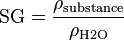# Specific gravity facts for kids

Kids Encyclopedia Facts

Specific Gravity (SG) is a special case of relative density.

It is defined as the ratio of the density of a given substance, to the density of water (H2O). Substances with a specific gravity greater than 1 are heavier than water, and those with a specific gravity of less than 1 are lighter than water.$\mbox{SG} = \frac{\rho_\mathrm{substance}}{\rho_\mathrm{H2O} }$

SG is by definition dimensionless and therefore not dependent on the system of units used (e.g. slugsft−3 or kgm−3), insofar as the units are consistent. Based on the SG-value of a given substance, the density of that substance can be calculated.

## Images for kidsSpecific gravity Facts for Kids. Kiddle Encyclopedia.